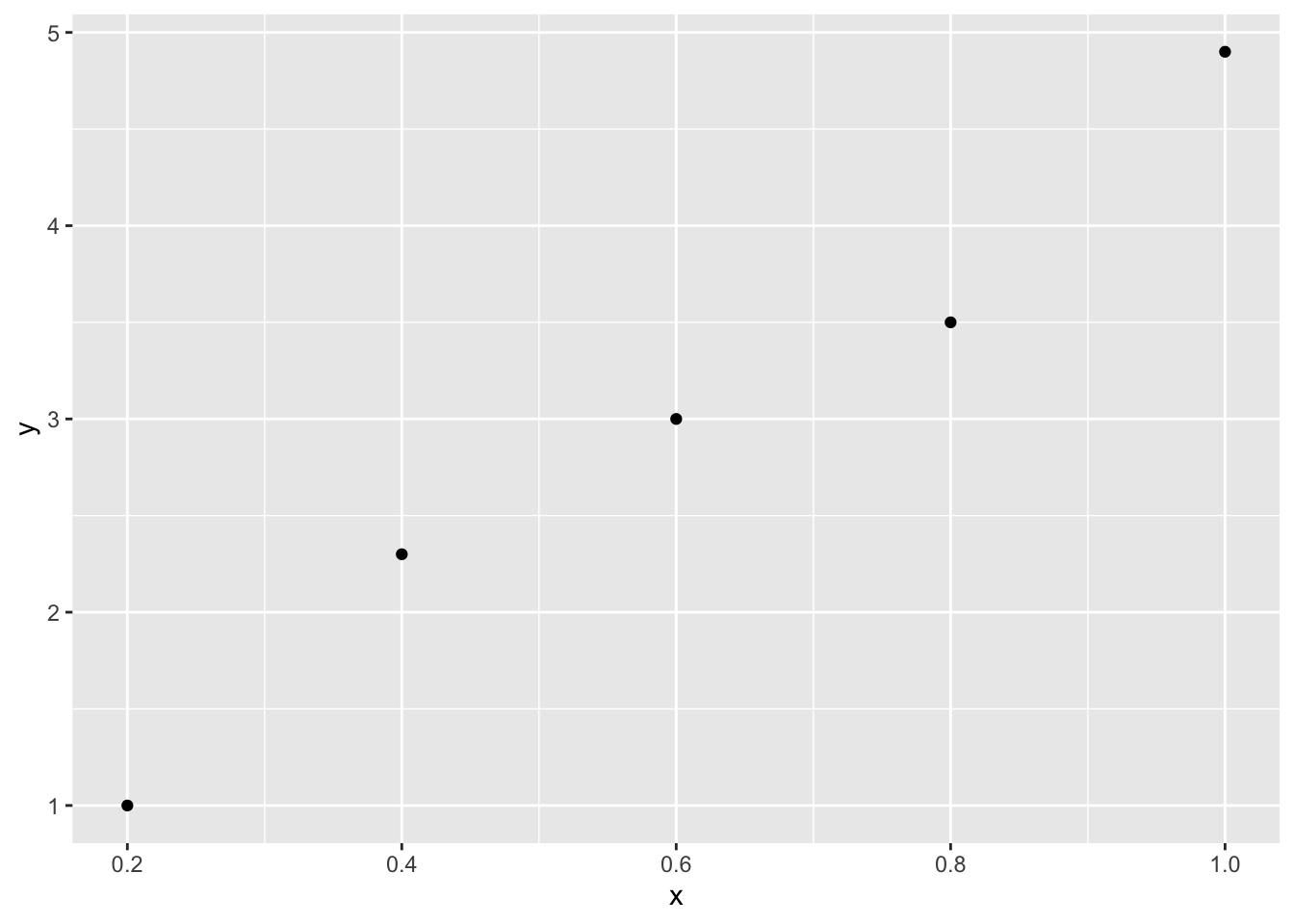### Definition

Given $(Y_1, X_1), (Y_2, X_2),\dots,(Y_n, X_n) \sim F_{Y, X}$ the goal is to obtain the regression function defined as

$r(x) = E[Y|X=x] = \beta_0 + \beta_1x$

#### Alternative definition

We have $$n$$ pairs of random variables in which each pair is

$Y_i = \beta_0 + \beta_1X_i + \epsilon_i$ with $E[\epsilon_i|X_i] = 0$

and

variance that does not depend on $$X_i$$

$V[\epsilon_i|X_i]=\sigma^2$

Sometimes, it is also assumed that the variability is normal

$\epsilon_i|X_i \sim N(0,\sigma^2)$

that is,

$Y_i|X_i \sim N(\mu_i, \sigma^2)$ where $$\mu_i = \beta_0 + \beta_1X_i$$.

### Fitted line

$\hat{r}(x) = E[Y|X]= \hat{\beta}_0+\hat{\beta}_1x$

### Predicted values

$\hat{Y}_i = \hat{r}(X_i)$

### Residuals

$\hat{\epsilon}_i = Y_i -\hat{Y}_i= Y_i - \left( \hat{\beta}_0+\hat{\beta}_1x \right)$

### Residuals sums of squares

$RSS = \sum_{i=1}^n \hat{\epsilon}_i^2$

### Least squares estimation

The least squares estimates $$\hat{\beta_0}$$ and $$\hat{\beta_1}$$ are the ones that minimize RSS.

#### Theorem

$\hat{\beta_1} = \frac{cov(X, Y)}{V(X)}$ $\hat{\beta_0} = \overline{Y}_n - \hat{\beta_1}\overline{X}_n$ Unbiased estimat of $$\sigma$$

$\hat{\sigma} = \frac{RSS}{n-2}$

#### Properties

Let

$X^n = (X_1, \dots, X_n)$ $\beta = \begin{bmatrix} \beta_0 \\ \beta_1 \end{bmatrix}$

Then,

$E \left( \hat{\beta} |X^n\right) = \begin{bmatrix} \beta_0 \\ \beta_1 \end{bmatrix}$

$V \left( \hat{\beta} |X^n\right) = \frac{\sigma^2}{n s^2_x} \begin{bmatrix} \frac{1}{n}\sum_{i=1}^n X_i^2 & -\overline{X}_n \\ -\overline{X}_n & 1 \end{bmatrix}$

where

$s^2_x = \frac{\sum_{i=1}^n (X_i - \overline{X}_n)^2}{n}$

The standard errors correspond to the diagonal terms of the matrix replacing $$\sigma$$ by $$\hat\sigma$$

$\hat{se}(\hat\beta_0 | X^n) = \frac{\hat\sigma}{s_x \sqrt{n}} \sqrt{\frac{\sum_{i=1}^nX_i^2}{n}}$ $\hat{se}(\hat\beta_1 | X^n) = \frac{\hat\sigma}{s_x \sqrt{n}}$

Consistency

$$\beta_0$$ and $$\beta_1$$ are consistent.

Asymptotic normality

$\frac{\hat\beta_0 - \beta_0}{\hat{se}(\hat\beta_0)} \leadsto N(0,1)$ $\frac{\hat\beta_1 - \beta_1}{\hat{se}(\hat\beta_1)} \leadsto N(0,1)$ Approximate $$(1-\alpha)$$ confidence intervals $\hat\beta_0 \pm z_{\alpha/2} \hat{se}(\hat\beta_0)$ $\hat\beta_1 \pm z_{\alpha/2} \hat{se}(\hat\beta_1)$ The Wald test for testing $H_0: \beta_1 = 0$ $H_1: \beta_1 \neq 0$ is rejecting $$H_0$$ if $$|W| > z_{\alpha/2}$$

where $W = \frac{\hat\beta_1}{\hat{se}(\hat\beta_1)}$

### MLE

#### Theorem

If

$\epsilon_i|X_i \sim N(0,\sigma^2)$

The MLE estimates for the intercept and the slope are the least squares estimates and

$\hat{\sigma} = \frac{RSS}{n}$

##### Demonstration

$L(\theta|x,y)=f(x,y|\theta)$

We don’t know the exact probability density functions for $$Y_1, Y_2,..,Y_n$$ , but we know that for each $$Y_i$$ the shape is the shape of the normal distribution centered around $$\mu_i = \beta_0+\beta_1x_i$$. Then, the probability density function for $$Y_i$$ is $f(x_i,y_i\mid\theta)=f(x_i,y_i\mid \beta_0,\beta_1,\sigma)=\frac{1}{\sqrt{2\pi\sigma^2}}e^{-\frac{(y_i-\beta_0-\beta_1x_i)^2}{2\sigma^2}}$

and the log likelihood $log(L(\beta_0,\beta_1,\sigma))=-\frac{n}{2} log(2 \pi \sigma^2) - \frac{1}{2 \sigma^2} \sum_{i=1}^{n} (y_i-\beta_0-\beta_1 x_i)^2$

It is clear that to maximize the likelihood we need to minimize the summation term, which actually corresponds to the least squares minimization. So the MLE intercept and slope corresponds to the typical regression model parameters. Introducing the best $$\beta_0$$ $$(\beta_{0MLE})$$ and $$\beta_1$$ $$(\beta_{1MLE})$$ and derivating relative to $$\sigma$$ is easy to find the MLE for $$\sigma^{2}$$ $\sigma_{MLE}^{2}=\frac{1}{n} \sum_{i=1}^{n} (y_i-\beta_{0MLE}-\beta_{1MLE} x_i)^2=\frac{RSS}{n}$

#### Example

library('ggplot2')
x<-c(.2,.4,.6,.8,1)
y<-c(1,2.3,3,3.5,4.9)
dat<-data.frame(x,y)

p<-ggplot()+
geom_point(data=dat,aes(x=x,y=y))
plogL<-function(p) {
n<-length(x) #A: p,  B: p,  sigma: p
-.5*n*log(2*pi*p^2)-1/(2*p^2)*sum((y-p-p*x)^2)
}
negLogL<-function(x) -logL(x)
MLEparameters<-optim(c(0,1,.1), negLogL)\$par
MLEparameters
##  0.2407149 4.4988915 0.2153094
xSeq<-seq(0,1,.1)
yPred<-MLEparameters+MLEparameters*xSeq
curveMLE<-data.frame(xSeq,yPred)
p+geom_line(data=curveMLE,aes(x=xSeq,y=yPred))We can calculate which is the likelihood for the best parameters

logL(MLEparameters)
##  0.5814404

Of course, we can estimate the parameters and the likelihood more directly

lm(y~x)
##
## Call:
## lm(formula = y ~ x)
##
## Coefficients:
## (Intercept)            x
##        0.24         4.50
logLik(lm(y~x))
## 'log Lik.' 0.5814469 (df=3)